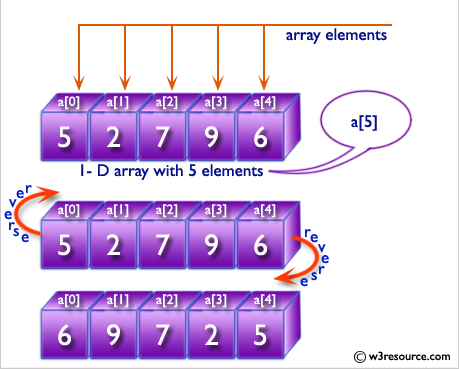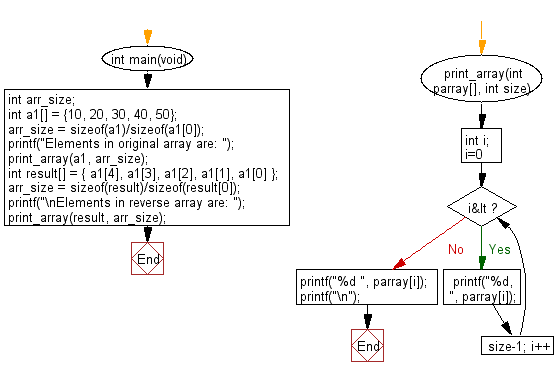﻿ C Program: Reverse a given array of integers and length 5 - w3resource# C Exercises: Reverse a given array of integers and length 5

## C-programming basic algorithm: Exercise-38 with Solution

Write a C program to reverse a given array of integers and length 5.

C Code:

``````#include <stdio.h>
#include <stdlib.h>
int main(void){
int arr_size;
int a1[] = {10, 20, 30, 40, 50};
arr_size = sizeof(a1)/sizeof(a1);
printf("Elements in original array are: ");
print_array(a1, arr_size);
int result[] = { a1, a1, a1, a1, a1 };
arr_size = sizeof(result)/sizeof(result);
printf("\nElements in reverse array are: ");
print_array(result, arr_size);
}
print_array(int parray[], int size)
{
int i;
for( i=0; i<size-1; i++)
{
printf("%d, ", parray[i]);
}
printf("%d ", parray[i]);
printf("\n");
}
``````

Sample Output:

```Elements in original array are: 10, 20, 30, 40, 50

Elements in reverse array are: 50, 40, 30, 20, 10
```

Pictorial Presentation:Flowchart:C Programming Code Editor:

What is the difficulty level of this exercise?

Test your Programming skills with w3resource's quiz.

﻿

## C Programming: Tips of the Day

Why doesn't a+++++b work?

printf("%d",a+++++b); is interpreted as (a++)++ + b according to the Maximal Munch Rule!.

++ (postfix) doesn't evaluate to an lvalue but it requires its operand to be an lvalue.

! 6.4/4 says the next preprocessing token is the longest sequence of characters that could constitute a preprocessing token"

Ref : https://bit.ly/3fdldUT